# Methods and formulas for goodness-of-fit statistics in Fit Regression Model

Select the method or formula of your choice.

## S

### Notation

TermDescription
MSEmean square error

## R-sq

R2 is also known as the coefficient of determination.

### Notation

TermDescription
yi i th observed response valuemean responsei th fitted response

While the calculations for adjusted R2 can produce negative values, Minitab displays zero for these cases.

### Notation

TermDescription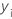ith observed response value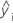ith fitted responsemean response
nnumber of observations
pnumber of terms in the model

## R-sq (pred)

While the calculations for R2(pred) can produce negative values, Minitab displays zero for these cases.

### Notation

TermDescription
yi i th observed response value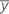mean response
n number of observations
ei i th residual
hi i th diagonal element of X(X'X)–1X'
X design matrix

## PRESS

Assesses your model's predictive ability and is calculated as:

### Notation

TermDescription
nnumber of observations
eiith residual
hi

ith diagonal element of

X (X' X)-1X'

## Test S

Test S summarizes the distance between the data values and the fitted values in the test data set. Test S is measured in the units of the response.

### Formula

where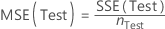.

For regression,

and for weighted regression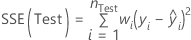.

### Notation

TermDescription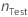number of rows in the test data set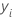ith observed response value in the test data setith fitted value for the response in the test data set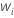weight for the ith observation in the test data set

## Test R-sq

Test R2 is the percentage of variation in the response variable of the test data set that the model explains. The value of test R2 ranges between 0% and 100%. (While the calculations for test R2 can produce negative values, Minitab Statistical Software displays 0 for these cases.)

### Formula

where for regression

and for weighted regression.

The formula for the total sums of squares also depends on whether the data include weights. For regression,

and for weighted regression
where

### Notation

TermDescriptionnumber of rows in the test data setith observed response value in the test data setith fitted value for the response in the test data setweight for the ith observation in the test data set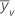mean of the response for the test data set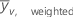weighted mean of the response for the test data set

## K-fold S

K-fold S summarizes the distance between the data values and the fitted values in the test data set. K-fold S is measured in the units of the response.

### Formula

where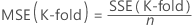.

For regression,

and for weighted regression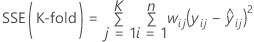.

### Notation

TermDescription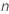number of rows in fold j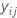ith observed response value in fold j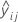ith cross-validated fitted value for the response in fold j
Knumber of folds
wiweight for the ith observation in fold j

## K-fold R-sq

K-fold R2 is the percentage of variation in the response variable of the data folds that the model explains. The value of K-fold R2 ranges between 0% and 100%. (While the calculations for K-fold R2 can produce negative values, Minitab Statistical Software displays 0 for these cases.)

### Formula

Minitab calculates the sum of squares for error for each fold. These calculations use the same model terms for every fold, but the estimates of the coefficients can differ. To calculate the k-fold R2 statistic, sum the sums of squares for error from the different folds. For regression

and for weighted regression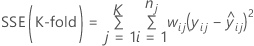.

Then, the following formula gives the equation for k-fold R2:

### Notation

TermDescription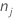number of rows without missing values for the response or missing values for the predictors that form the candidate terms in the modelith observed response value in fold jith cross-validated fitted value for the response in fold j
Knumber of folds
wijweight for the ith observation in fold j
SSTotaltotal sum of squares for all of the data

## K-fold stepwise R-sq

K-fold stepwise R-sq evaluates the number of terms in a model from a set of candidate terms. Minitab displays negative values for k-fold stepwise R-sq when they occur.

### Formula

Minitab calculate k-fold stepwise R-sq when the stepwise selection method is forward selection with validation and the validation method is k-fold cross-validation. Minitab performs forward selection K times, omitting the data for each fold once. The model for each fold can be different. Once the forward selection procedures are complete, Minitab sums the squared errors for all folds at each step. Minitab uses this sum to calculate k-fold stepwise R-sq. For regression:

and for weighted regression: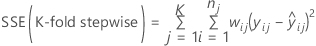Then, the following formula gives the k-fold stepwise R2 value for a step.

### Notation

TermDescriptionnumber of rows without missing values for the response or missing values for the predictors that form the candidate terms in the modelith observed response value in fold jith cross-validated fitted value for the response in fold j
Knumber of folds
wijweight for the ith observation in fold j
SSTotaltotal sum of squares for all of the data

## Log-likelihood

For unweighted analyses, Minitab uses the following equation:
For an analysis that has weights for the observations, Minitab uses the following equation:

Observations with weights of 0 are not in the analysis.

### Notation

TermDescription
nthe number of observations
Rthe sum of squares for error for the model
withe weight of the ith observation

## AICc (Akaike's Corrected Information Criterion)

AICc is not calculated when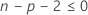.

### Notation

TermDescription
nthe number of observations
pthe number of coefficients in the model, including the constant

## BIC (Bayesian Information Criterion)

### Notation

TermDescription
pthe number of coefficients in the model, including the constant
nthe number of observations

## Mallows' Cp

### Notation

TermDescription
SSEpsum of squared errors for the model under consideration
MSEmmean square error for the model with all candidate terms
nnumber of observations
pnumber of terms in the model, including the constant
By using this site you agree to the use of cookies for analytics and personalized content.  Read our policy